### SAT数学：圆的方程 2

2019-09-12 SAT-数学 阅读

# SAT Question Rank: Medium Topic: Algebra, Geometry, Equation of a Circle

Below is a question from a recent SAT exam. It is a non-calculator question that would typically take a student 1-2 minutes to complete.

A circle in the xy-plane has center (5, 7) and radius 2. Which of the following is an equation of the circle?

(A)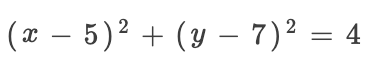(B)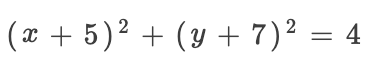(C)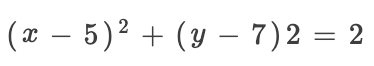(D)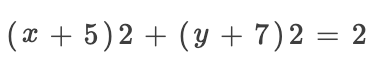To begin, we will recall the formula for the equation of a circle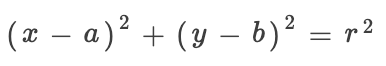Where a is the x-coordinate for the centre of the circle, b is the y-coordinate of the centre of the circle, and r is the radius.
We are told in the question that the circle has a radius of 2. Therefore,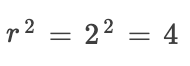Our equation now looks like this.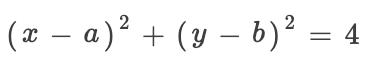Next, we substitute a and b for the values given as the circle's centre.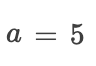and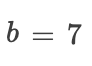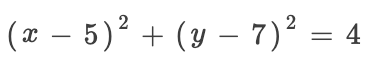• 地址：401-5811 Cooney Rd, Richmond, BC
• 地址：3030-8171 Ackroyd Rd, Richmond, BC
• 客服热线：604-370-0579 & 604-370-2569
• 联系邮箱：service@forbest.com

• 地址：江苏省无锡市梁溪区清扬路268号三楼
• 客服热线：181-1861-8855

•官方公众号
•加拿大官方客服
•中国官方客服